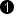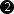## Operator FIRoperatorNxM

The operator FIRoperatorNxM calculates the sum of the multiplication of the input kernel elements with parameterizable coefficients. The coefficients can be defined using parameter Coefficients.

Lets have a look at an example. Assume the following Sobel filter coefficients H to detect vertical edges

This filter is applied to the following image I

For the pixel at position I(2,2) = 31 we get the following result at output O

To generate the required input kernel use operators such as FIRkernelNxM, LineNeighboursNx1 or PixelNeighbours1xM.

Operator Restrictions

• ImageWidth < 2*Parallelism are not allowed

• Empty images i.e. images with no pixels are not allowed.

• Empty lines or varying line lengths are not allowed.

### I/O Properties

Property Value
Operator Type O

Bit Width [1, 63] unsigned, [2, 63] signed autoArithmetic {unsigned, signed} autoParallelism any as I
Kernel Columns any 1
Kernel Rows any 1
Img Protocol {VALT_IMAGE2D, VALT_LINE1D, VALT_PIXEL0D} as I
Color Format VAF_GRAY as I
Color Flavor FL_NONE as I
Max. Img Width any as I
Max. Img Height any as I
 The required output bit width is automatically determined from the input bit width, arithmetic and coefficients. The output bit width must not exceed 64 bit. The output arithmetic is automatically determined from the input arithmetic and the coefficients. The output is signed if either the input is signed or at least one coefficient is signed.

### Parameters

Coefficients
Type static parameter
Default identity
Range

This parameter defines the coefficients of the filter kernel. Signed and unsigned integer values are allowed.

If the input kernel size of the operator is changed, the coefficients will also change. Check the coefficients after changing the input kernel size.

The coefficient values may not cause the output bit width to exceed 64 bit.

### Examples of Use

The use of operator FIRoperatorNxM is shown in the following examples: